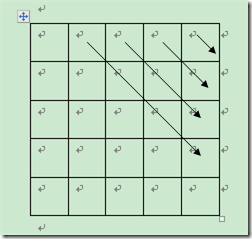## 《编程珠玑，字字珠玑》45678读书笔记——编程技巧

2012-04-06 21:03  捣乱小子  阅读(...)  评论(...编辑  收藏

### 实战演练：动态规划矩阵连乘最优组合

“大概”伪代码：

for i=[0,n-1
for j=[0,n-1-i)
col =...    //col是填表元素的列
min =...
for k=[0,i)
t =....
if t<min
t = min
a[j][col] = min;

### 捣乱的分析过程for i=[0,n-1
for j=[0,n-1-i)

min = a[j][j] + a[j+1][col] + tab[j]*tab[j+1]*tab[col];

t = a[j][j+k+1] + a[j+k+2][col] + tab[j] * tab[j+k+2] * tab[col+1];View Code
void optimal_matrix(int * tab,int n)

assert(n!=0);
int ** a = new int *[n];
for(int i=0; i<n; i++)
a[i] = new int[n],
::memset(a[i],0,sizeof(int)*n);
int i,j,t,min,col;
for(i=0; i<n; i++)
a[i][i] = 0;
for(i=0; i<n-1; i++)//组计数器
{
for(j=0; j<n-1-i; j++)//每组个数计数器
{
col = i + j + 1
assert(col+1<n+1);
min = a[j][j] + a[j+1][col] + tab[j] * tab[j+1] * tab[col+1];
for(int k=0; k<i; k++)
{
assert(j+k+2<n);
t = a[j][j+k+1] + a[j+k+2][col] + tab[j] * tab[j+k+2] * tab[col+1];
if(t<min) min = t;
}// for
a[j][col] = min;
}// for
}// for
cout << a[n-1] << endl;
delete [] a;

### 断言的魅力

“脚手架”简单来说是“验证程序”的程序，但笔者认为“断言”的魅力更大些。在每一个程序中，有一些变量数据是至关重要的，经常Debug就是为了这些变量的检测，看是否和预期中的结果一样；如果不一样我的做法就是：结束debug，开始艰苦的错误排查，这个过程非常头疼。assert能够可以扫清很多的错误细节，包括除数为0，数据超出规定的范围，数组下标越界云云。所以添加断言，能在逻辑上保证你的程序不会出错，即使现实并非如此。

assert(n!=0);
assert(col+1<n+1);
assert(j+k+2<n);

void show(int ** a,int n)

for(int i=0; i<n; i++)
{
for(int j=0; j<n; j++)
cout << setw(6) <<a[i][j];
cout << endl;
}// for
cout << endl;
}

### 一个算法题

1. 最原始穷举的算法时间开销大到O(n^3)；
2. 另一种穷举的算法，即平方算法。通过保存中间结果或者预处理数据，省去了之后重复的计算，是备忘录算法（简单的动态规划），开销下降了一个数量级O(n^2)；
3. 分治法，这个真没想到，开销再次下降O(nlogn)；
4. 以前做过类似的题目，所以最先想到的就是这个方法。形象点就是，“边吃边拉”——扫描算法，运行时间为O(n)。
for i=[0,n)
t += a[i]
if t<max case
max = t
if t<0 case
t = 0
end.

ACM初赛的题目用的就是这个“边吃边拉”，不过和这里的有点不同，实际上是贪心算法：http://acm.hdu.edu.cn/diy/contest_showproblem.php?pid=1005&cid=14855&hide=0

nearest = INF
for i=[0,n)
for j=[i,n)
t = tab[j] - tab[i]
if nearest>|t| case
nearest = t
end

### 数组用负数索引

bubble(a,n)
mustbe(n>1
pa = &a[1
for i=[0,n)
for j=[0,n-i)
if a[j]>pa[j] case
swap(a[j],pa[j])
end

sum(a,n)
for i=[0,n)
sum += a[i];
end

sum(a,n)
pa = &a[n-1
t = n>1
for i=[0,t)
sum += (a[i]+pa[i|0x8000])    //下标变为负数
if n&1 case
sum += a[t]
end